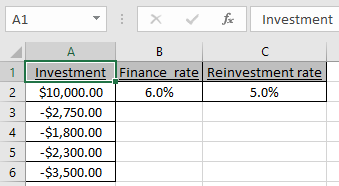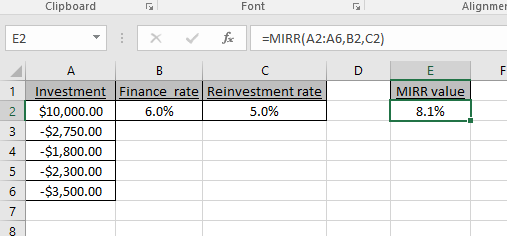# How to use the MIRR function in Excel

MIRR function returns the Modified interest rate of return for the financial data having Investment, finance rate & reinvestment_rate.

The MIRR function returns modified interest rate of return in percentage.
Syntax:

=MIRR (values, finance_rate, reinvest_rate)

Values : Investment amount (both positive & negative amount) or cash flows in array.
Finance_rate : Required rate of return (discount rate) as a percentage.
Reinvest_rate : Interest rate received on cash flows reinvested as percentage.

Let’s understand this function using it in an example.

Here we have Investment, Finance_rate & reinvestment_rate.Investment or cash flow array must contain both positive & negative amounts.

Use the formula:

=MIRR(A2:A6, B2, C2)

A2:A6 : investment amount or cash flow
B2 : finance_rate
C2 : Reinvest_rateAs you can see the MIRR function returns the modified interest rate of return is 8.1%.
Hope you understood how to use the MIRR function to get modified interest rate of return in Excel. Explore more articles on Excel financial functions here. Please feel free to state your query or feedback for the above article.

Related Articles:

How to use the DURATION Function in Excel

Excel IPMT Function

How to Use Compound Interest Function in Excel

Popular Articles:

How to use the VLOOKUP Function in Excel

How to use the COUNTIF function in Excel 2016

How to Use SUMIF Function in Excel

Terms and Conditions of use

The applications/code on this site are distributed as is and without warranties or liability. In no event shall the owner of the copyrights, or the authors of the applications/code be liable for any loss of profit, any problems or any damage resulting from the use or evaluation of the applications/code.